# Estimating the Laplace-Beltrami Operator by Restricting 3D Functions

SGP 2009 Paper
Windows Executables Source Code
Version 1 Page

CODE DESCRIPTION
This distribution provides an implementation of an algorithm for performing texture-stitching on meshes. Given a base model and a set of textured scans that have been registered to the model, a color gradient field is defined over the base mesh, its divergence is computed, and a Poisson system is solved to return the color field that best fits the gradients. We support a formulation with soft constraints, solving for the funciton f that minimizes: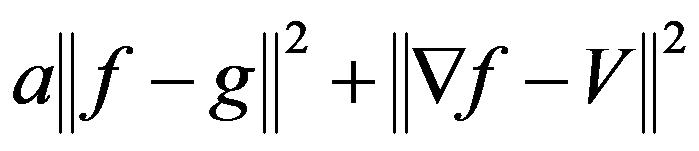where g is the color field extracted from the scans, V is the vector field of gradients obtained from the scans, and a is the weighting term determing the importance of the value-interpolation constraints.
This implementation supports the specification of the scans both as rang-grids (which facilitates the look-up of gradient information) and as meshes with color vertex (requiring a less efficient kD-tree query to obtain the gradient information).

EXECUTABLE ARGUMENTS
--in <input base mesh>
This string is the name of the file from which the base mesh will be read. The file is assumed to be in PLY format.
--scans <input scans>
This string is the name of the file containing the list of scans. Each scan is assumed to be in PLY format.
--out <output mesh>
This string is the name of the file to which the seamlessly textured mesh will be written. The mesh will be written out in PLY format, and the colors will be encoded as vertex properties of type unsigned char with names red/green/blue.
[--useKD]
If this optional parameter is specified, the input scans are read in as meshes and the nearest point to a sample on a base mesh is found using a kD-tree. Otherwise, the scans are in range-grid format, and finds the scan value by back-projection.
[--xForms <transform list>]
This optional string is the name of the file listing the names of the transformations (each encoded separately as an ASCII file with 4x4 matrix entries) that register the scans to the base mesh. It is assumed that there are the same number of transformations as there are scans, and that the listing is given in the same order.
[--depth <maximum octree depth>]
This optional integer specifies the maximum depth of the tree that will be used for defining the finite-elements system. Running at depth d corresponds to solving on a voxel grid whose resolution is no larger than 2^d x 2^d x 2^d. The default value for this parameter is 8.
[--primal]
If this optional argument is specified, the system is defined using first-order elements, which have smaller support and therefore define a sparser system with lower dimension. Otherwise, second-order elements are used.
[--iters <Gauss-Seidel iterations>]
This optional integer specifies the number of iterations of Gauss-Seidel relaxation that are to be performed at each level of the multigrid solver. The default value for this parameter is 10.
[--cycles <solver cycles>]
This optional integer specifies the number of full multigird cycles that are to be run in solving the linear system. The default value for this parameter is 1.
[--monteCarlo <integration samples>]
This optional integer specifies the number of point samples that are to be used to approximate the integrals defining the system coefficients. If the parameter is not specified, Taylor's asymmetric cubature formula is used to explicitly compute the values of the integrals.
[--vWeight <value weight>]
This optional floating point value specifies the weight given to the constraint that resulting texture should be a good fit to the color values of the scans. The default value for this parameter is 0.0.
[--subdivide <triangle subdivision iterations>]
This optional integer specifies the number of times the triangles of the mesh are to be subdivided. (Larger values results in a finer mesh, that more densely samples the reconstructed color functions, and more accurately captures the high-frequency detail, but requires more time and space for computation). The default value for this parameter is 0.
[--scale <scale factor>]
This optional floating point value specifies the ratio between the diameter of the cube used for reconstruction and the diameter of the meshs' bounding cube. The default value is 1.1.
[--grazingFallOff <fall-off exponent>]
This optional floating point value specifies the exponent used to modulate distance priorities to down-play the contribution of scan points at grazing angles. The defaulf value is 2.0.
[--noSolver]
If this optional argument is specified, the code does not solve the linear system, but instead returns a mesh that is textured by grabbing the color from the nearest scan.
[--ascii]
If this optional argument is specified, the output .ply file is written in ascii format. Otherwise, the output is written in binary.
[--double]
If this optional argument is specified, the system is defined and solved using double-precision arithmetic. Otherwise, single precision is used.
[--verbose]
If this optional argument is specified, the code outputs information about running and time and memory usage througout the processing.
[--progress]
If this optional argument is specified, the code shows a progress bar for the different steps of the system set-up.
[--noTSamples]
[--noRSampling]
By default, if Monte-Carlo sampling is used, the point samples are enriched by adding four points (uniformly sampled) from each triangle. This attempts to ensure that each vertex falls into an existing octree node at the finest resolution, so that values at vertices are well-defined.
[--noRSampling]
If this optional flag is enabled, only one point-per-triangle is used for augmentation.
[--noTSamples]
If this optional flag is enabled the point samples used for estimating the integrals are not augmented at all.

USAGE
For testing purposes, three datasets are provided.
1. Rooster: This dataset, obtained using the NextEngine scanner, consists of 8 scans that are represented in range-grid format.
To obtain the seamlessly textured surface, we execute:
``` TextureStitcher --in rooster.ply --scans scans.txt --out out.ply --xForms xForms.txt ```
To reconstruct the finer details we can subdivide the mesh in order to sample the gradient field more densely:
``` TextureStitcher --in rooster.ply --scans scans.txt --out out.ply --xForms xForms.txt --subdivide 1 ```
We can also reduce the time required to set up and solve the linear system by using first-order elements and approximating the integrals by a discrete summation:
``` TextureStitcher --in rooster.ply --scans scans.txt --out out.ply --xForms xForms.txt --subdivide 1 --primal --monteCarlo 5000000 ```
Finally, if we would like to prevent color-bleeding due to the missing gradients at the bottom of the model, we can add in a soft constraint on the values:
``` TextureStitcher --in rooster.ply --scans scans.txt --out out.ply --xForms xForms.txt --subdivide 1 --primal --monteCarlo 5000000 --vWeight 0.01 ```
A visualization of the results can be seen in the images below. (The left-most image shows the results obtained when the colors are obtained by directly copying the color values from the nearest scan.)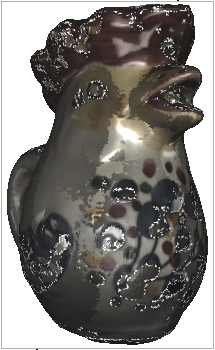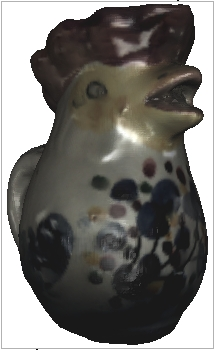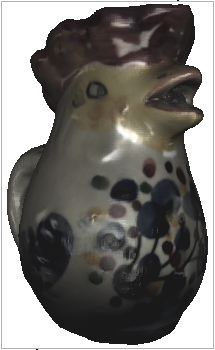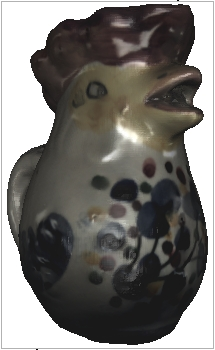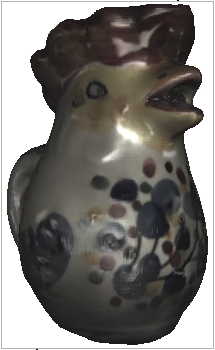2. Male04: This dataset, obtained courtesy of Cyberware scanner, consists of 4 scans that are represented as triangle meshes with color values associated to each vertex.
To obtain the seamlessly textured surface, we execute:
``` TextureStitcher --in male04.base.ply --scans male04.scans.txt --out out.ply --useKD --depth 10 --primal --monteCarlo 5000000 ```
To correct for the loss of gradients near the sleeve that result in color-bleed through to the arm, we introduce a soft constraint on the values:
``` TextureStitcher --in male04.base.ply --scans male04.scans.txt --out out.ply --useKD --depth 10 --primal --monteCarlo 5000000 --vWeight 0.01 ```
A visualization of the results can be seen in the images below. (The left-most image shows the results obtained when the colors are obtained by directly copying the color values from the nearest scan.)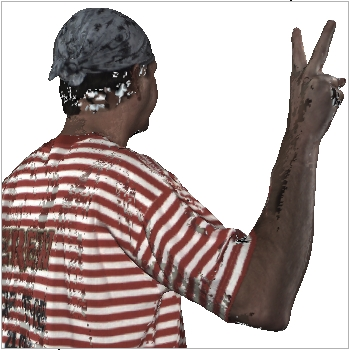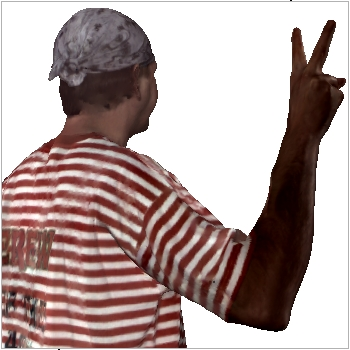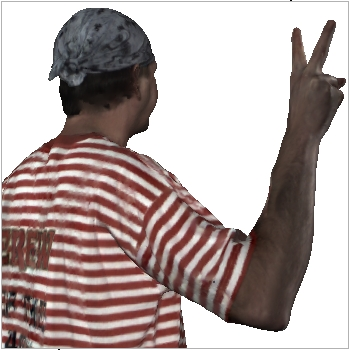3. Pleo: This dataset of the pleo robot, obtained using a Minolta Vivid 910 scanner, and generously contributed by Mario Botsch, consists of 20 scans that are represented as triangle meshes with color values associated to each vertex.
To obtain seamlessly textured surfaces with varying interpolation weights, we execute:
``` TextureStitcher --in Pleo.base.ply --scans Pleo.scans.txt --out out.ply --useKD --depth 10 --primal --monteCarlo 5000000 --subdivide 1 --vWeight <10/0.1/0.001/0.00001> ```
A visualization of the results can be seen in the images below.
--vWeight 10 --vWeight 0.1 --vWeight 0.001 --vWeight 0.00001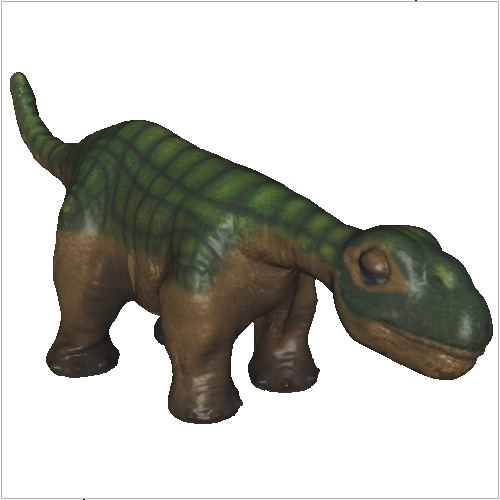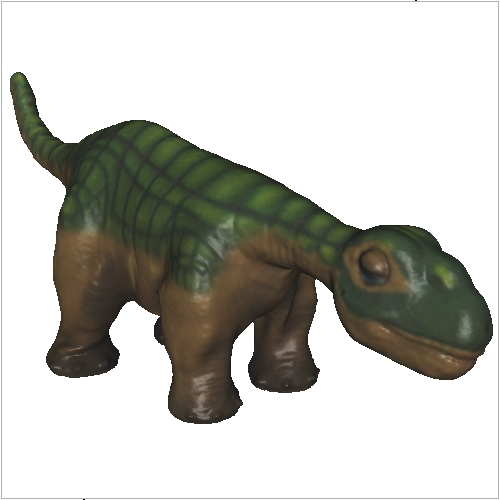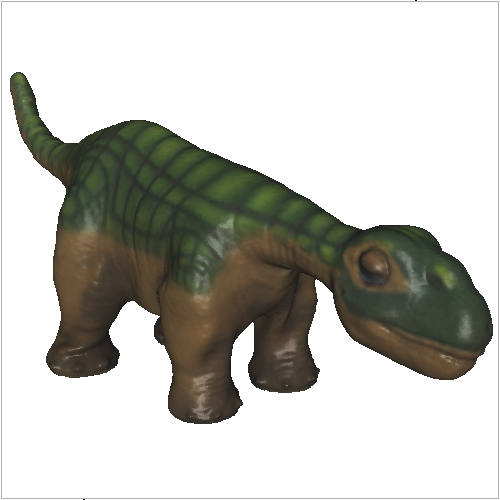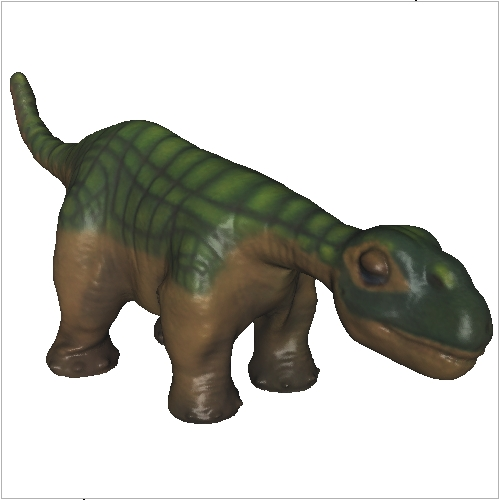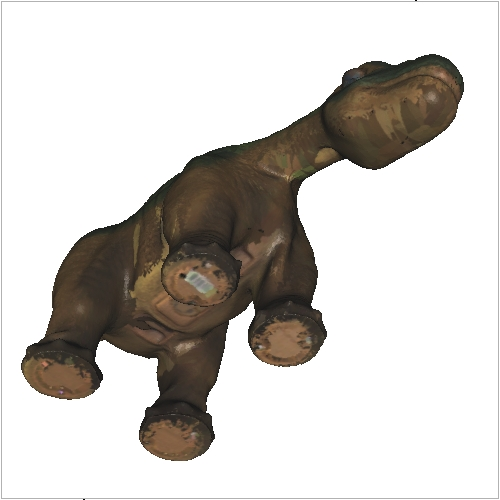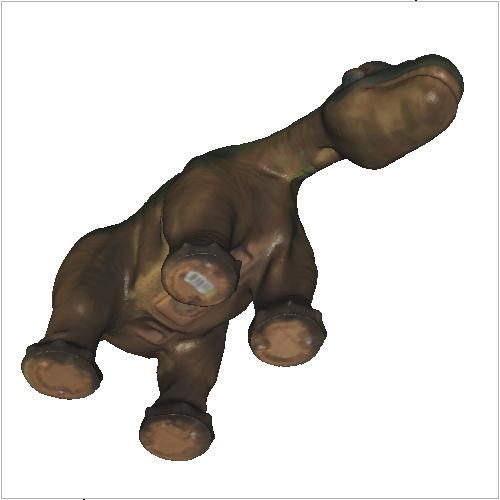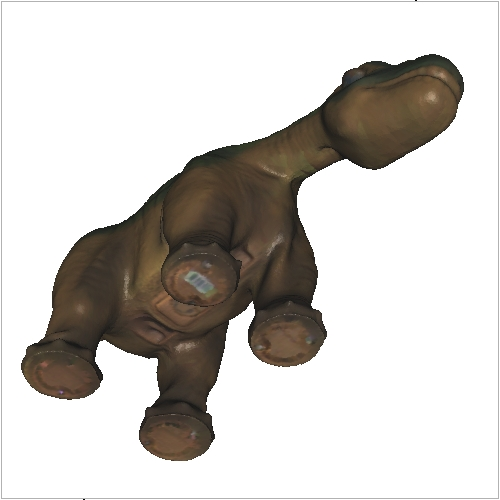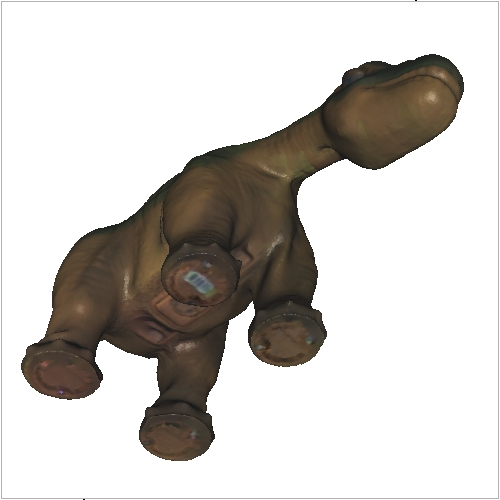HOME